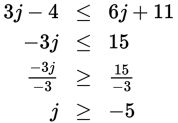# SAT Math Multiple Choice Question 811: Answer and Explanation

### Test Information

Question: 811

1. Which of the following gives all values of j that satisfy the inequality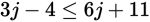?

• A.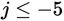• B.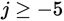• C.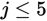• D.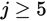Explanation:

B

Difficulty: Easy

Category: Heart of Algebra / Inequalities

Strategic Advice: When an inequality has only one variable, straightforward algebra is the best route to the answer.

Getting to the Answer: Solve for j by moving the variable terms to one side of the inequality and the constants to the other, remembering to flip the inequality symbol if you multiply or divide by a negative number: